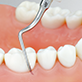### Perio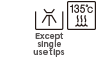•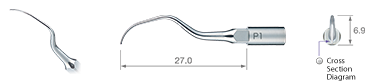• Model:P1
• Order Code:Z217404
•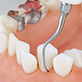•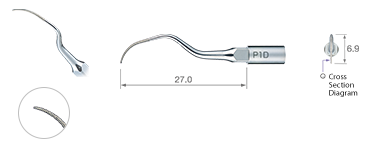• Model:P1D
• Order Code:A1012972

Diamond coated / Single use

•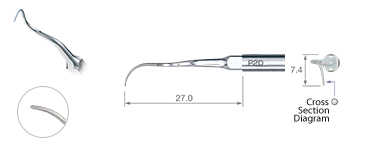• Model:P2D
• Order Code:A1012970

Diamond coated / Right curved type / Single use

•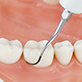•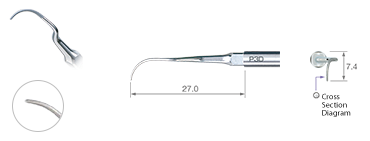• Model:P3D
• Order Code:A1012971

Diamond coated / Left curved type / Single use

•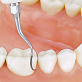•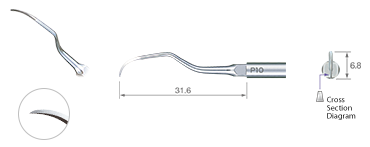• Model:P10
• Order Code:Z217410

Sharp edge

•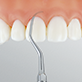•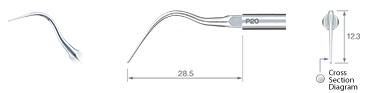• Model:P20
• Order Code:Z217420
•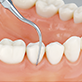•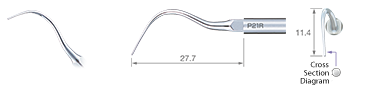• Model:P21R
• Order Code:Z217422

Right curved type

•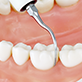•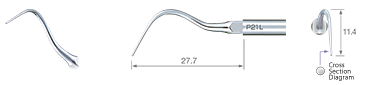• Model:P21L
• Order Code:Z217421

Left curved type

•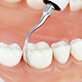•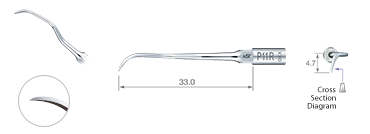• Model:P11R
• Order Code:Z217408

Sharp edge / Right curved type

•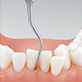•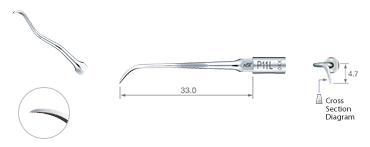• Model:P11L
• Order Code:Z217409

Sharp edge / Left curved type

•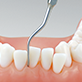•• Model:P25R
• Order Code:Z217424

Right curved type

•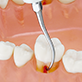•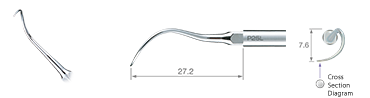• Model:P25L
• Order Code:Z217425

Left curved type

•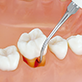•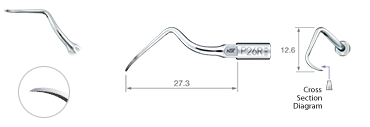• Model:P26R
• Order Code:Z217426

Right curved type

•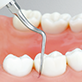•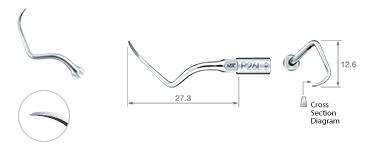• Model:P26L
• Order Code:Z217427

Left curved type

•